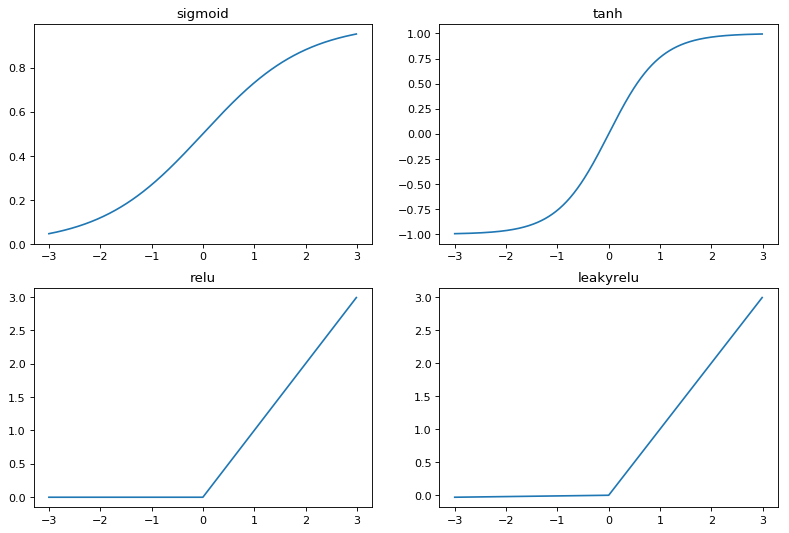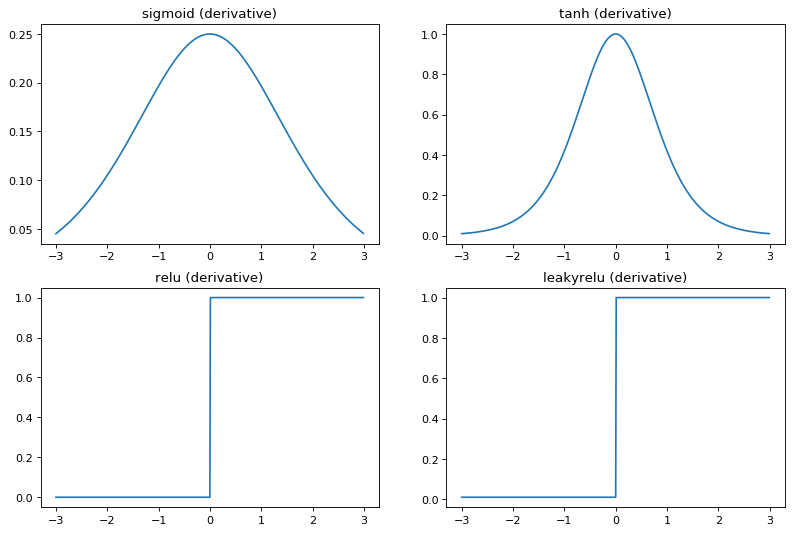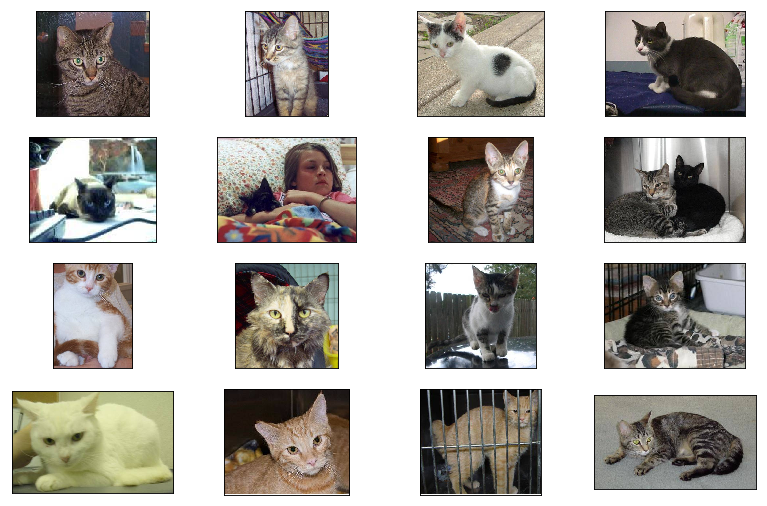Part of ML notes: Neural Networks and Deep Learning

# Neural Networks (NNs)¶

Warning: This is only meant to illustrate the concept behind neural networks. In actual usage, use mature libraries like Tensorflow, PyTorch, Theano or such.

Neural networks are the bedrock of deep learning. Even though neural networks have been around for a long time, it is only recently, with the increase in parallel computing capabilities (with GPUs and parallel CPUs) and dataset sizes, that neural networks have started to outperform other forms of machine learning algorithms.
At its core, NN is a bunch of logistic regression units stacked recursively. Each layer of such units, termed neurons, feed into a next layer of them. Such an arrangement is capable of approximating any continuous function (see here for details). Rather than manually figuring out which higher-order features to include or not, this way of assigning multiple hidden layers does, in a given sense, automatic feature extraction. This may be why the neural network works remarkably well over a wide variety of tasks.

### Conventions¶

Identify a $L$-layer NN with weights $w_\alpha^{[l]}$ and bias $b^{[l]}_\alpha$, $1 \leq l \leq L$. Let $l=0$ represent the input/features layer, $l=L$ the output layer. The following functions are considered:
Sigmoid function. $$g(z)=\frac{1}{1+e^{-z}}$$ From standard calculus, $g'(z)=g(z)\{1-g(z)\}$.
tanh function. $$g(z)=tanh(z)=\frac{e^{z}-e^{-z}}{e^{z}+e^{-z}}$$ Again, it is a well known result that $g'(z)=1-g(z)^2$.
Restricted linear unit (ReLU) function. $$g(z)=max(0,z)$$ Here, $g'(z) = 1$ for $z>0, 0$ for $z<0$.
Leaky ReLU function. $$g(z)=max(\gamma z,z)$$ where $\gamma << 1$. Here, $g'(z) = 1$ for $z>0, \gamma$ for $z<0$.

In :
import numpy as np # linear algebra
import scipy as sp
import pandas as pd # data processing, CSV file I/O (e.g. pd.read_csv)
import cv2
import os
import matplotlib.pyplot as plt

In :
# define the different choice of activation functions
def activation(activationType, z):
if activationType == 'sigmoid':
return 1/(1+np.exp(-z))
elif activationType == 'tanh':
return np.tanh(z)
elif activationType == 'relu':
return (z>0)*z
elif activationType == 'leakyrelu':
return ((z>0)*z)+((z<0)*0.01*z) # choose gamma = 0.01

def activationDerivative(activationType, z):
if activationType == 'sigmoid':
g = activation('sigmoid', z)
return g*(1-g)
elif activationType == 'tanh':
g = activation('tanh', z)
return 1-(g**2)
elif activationType == 'relu':
return (z>0)
elif activationType == 'leakyrelu':
return (z>0)+((z<0)*0.01)

# plot them for intuition
activationList = ['sigmoid', 'tanh', 'relu', 'leakyrelu']
points = np.arange(-3,3,0.01)
plt.figure(num=None, figsize=(12, 8), dpi=80, facecolor='w', edgecolor='k')
for i, activationType in enumerate(activationList):
plt.subplot(2, 2, i+1)
plt.plot(points,activation(activationType, points))
plt.title(activationType)
plt.show()In :
points = np.arange(-10,10,0.01)
plt.figure(num=None, figsize=(12, 8), dpi=80, facecolor='w', edgecolor='k')

activationList = ['sigmoid', 'tanh', 'relu', 'leakyrelu']
points = np.arange(-3,3,0.01)
plt.figure(num=None, figsize=(12, 8), dpi=80, facecolor='w', edgecolor='k')
for i, activationType in enumerate(activationList):
plt.subplot(2, 2, i+1)
plt.plot(points, activationDerivative(activationType, points))
plt.title(activationType+' (derivative)')
plt.show()

<Figure size 960x640 with 0 Axes>In :
class NN:
def __init__(self, X, y, layersList=[(24, 'relu'), (24, 'relu')]):
# check dimension
if not (X.shape == y.shape):
raise Exception('Not enough labels:', 'Number of samples are ', X.shape, ' while number of labels are ', y.shape, '.')
elif not (y.shape == 1):
raise Exception('More than one label per sample.')
else:
# add an extra line of ones for theta_0
self.X = np.ones([X.shape, X.shape+1])
# do the allocation
self.X[:, 1:] = X
# different convention followed for NNs
self.X = X.T
self.y = y


### Forward propagation¶

For any layer $l \neq 0$, $a^{[l]} = g^{[l]}(z^{[l]})$, $z^{[l]} = \sum _\beta w^{[l]}_{\alpha \beta} a_\beta^{[l-1]}+b_\alpha^{[l]}$. This gives a recursive relation to compute $\hat{y}$ as follows: $$\hat{y} = g^{[L]}(z^{[L]}) = g^{[L]}(\sum_\beta w_{\alpha \beta}^{[L-1]}g^{[L-1]}((...)+b_\alpha^{[L-1]}))$$ This is basically the hypothesis of our neural network. The computation of the hypothesis function is usually termed the forward propagation, since the recursive relation starts from the input, and proceeds in the forward direction of the layers to yield $\hat{y}$.

In :
def fprop(self, w, b):
# check if dimensions of w, b are correct
a = self.X
for l, activationType in self.layerList:
self.z[l] = np.dot(self.weights[l-1], self.X) + b
a[l] = activation(activationType, z[l])

setattr(NN, 'fprop', fprop)

# model = NN(X, y, [(24, 'relu'), (10, 'relu'), (10, 'relu'), (1, 'sigmoid')])


### Backward propagation¶

This is nothing more than the chain rule for the derivative of the cost function. Note that for any $l \neq L$, $da^{[l]}=g'^{[l]}(z^{[l]})dz^{[l]}$, $dz^{[l]}=w^{[l]}_{\alpha \beta}da _\beta^{[l-1]}$

In :
def bprop(self, dw):
da[L] = dJ
for l, activationType in reverse(self.layerList):
self.da[l-1] = activationDerivative(activationType, self.X)

setattr(NN, 'bprop', bprop)


Gradient checking: Since the above algorithm is quite involved, it helps to know if your implementation is actually working or not. A simple way to check this is to use gradient checking. Further down the line, we will encounter more sophisticated architectures where the backpropagation can get even more complicated. The method described helps a great deal to convince us that our implementations are indeed working fine.

1. Problem of local extrema: In the case of NNs, the non-convexity of the cost function means that local minima is not necessarily the global one. Saddle points and plateaus are more common than extrema. Gradient with momentum, RMSProp, Adam, He initialising

2. Problem of vanishing/exploding gradient: The initial value of weights is quite important, otherwise the gradient descent may take forever to converge to the minima. To see this let us take a very deep ($L=10$) network.

3. Symmetry: Initialising each parameter to the same quantity

4. Large datasets:

### Image classification: cats vs. dogs¶

In :
# loading cat images
cat_dict = {}
cat_dict[filepath[4:-4]] = cv2.cvtColor(cat_dict[filepath[4:-4]], cv2.COLOR_BGR2RGB)

In :
import matplotlib.pyplot as plt
import matplotlib.image as mpimg
plt.figure(num=None, figsize=(12, 8), dpi=80, facecolor='w', edgecolor='k')
for i in range(16):
plt.subplot(4, 4, i+1)
plt.tick_params(axis='both',
which='both',
bottom=False,
top=False,
labelbottom=False,
right=False,
left=False,
labelleft=False)
plt.imshow(cat_dict[str(i+1)])Until now, the NNs covered had a specific type of architecture: entire layers that connected to all the neurons in the next layer, and so on. This is actually a specific type of NN called the feed-forward NN. Depending on the problem at hand, different types of architectures can be used that is more suited to the problem at hand.
For instance, for computer vision related tasks, it makes sense that we make the architecture sensitive to the spatial nature of the task; similarly so for sequential data. There is most certainly a sense of intuition in choosing these architectures, but a lot comes from trying new models and seeing how they perform. In this respect, two versions called the convolutional NN (CNN) and recurrent NN (RNN) are well suited, especially for computer vision and NLP respectively.

## Convolutional Neural Networks (CNN)¶

When you feed an image into a NN, each pixel goes in as input separately. But this is not how we view images; we often have a sense of spatial sense of the image. At the very first layer, instead of taking input from all the inputs, we can localise feature selection by taking the neighbouring pixels, and repeatedly using this technique uniformly at each point. The operation we are looking at is convolution. In reality, it is a combination of convolution and pooling when combined over multiple layers that work best for image classification and other related tasks. The main operations are as follows:

1. Convolution. Given an input matrix $M$ of dimension $n_h \times n_v$, and a square matrix $F$ called the filter/kernel matrix of dimension $f \times f$) s.t. $n_w,n_h \leq f$, the convolution $M*F$ is a $(n_w -f + 1) \times (n_h -f + 1)$ matrix given as follows: $$(M*F)_{\alpha \beta} := \sum _{\substack{\alpha \leq \gamma \leq \alpha + f - 1 ,\\ \beta \leq \delta \leq \beta + f - 1}} M_{\gamma \delta} F_{\gamma \delta}$$
The procedure here is quite intuitive when imagined as block of $M$ multiplied pointwise with $F$ to form a smaller matrix.
3. Pooling.
• Max pooling
• Average pooling
In :
# this function multiplies the larger image matrix with the individual elements of the filter matrix
# this should be faster than the standard other way around
# sure I'm not the only one who thought of this
def conv2D(image, filter_matrix, stride):
# stride not implemented yet
f = filter_matrix.shape
output_size = tuple(np.array(image.shape) - np.array([f-1,f-1,0]))
X = np.zeros(output_size, dtype='uint8')
for i in range(f):
for j in range(f):
for ch in range(filter_matrix.shape):
X[:,:,ch] = X[:,:,ch] + (filter_matrix[i,j,ch] * image[i:i+output_size, j:j+output_size, ch])
return np.sum(X, axis=2)

# check image is in right format
if not (len(image.shape) == 3):
raise Exception('Image not in the right format! Needs to be a numpy array of 3 dimensions')
X = 255*np.ones(shape, dtype='uint8')
return X

def maxPool(image, filter_size, stride):
# stride not implemented yet
f = filter_size
output_size = tuple(np.array(image.shape) - np.array([f-1,f-1,0]))
X = np.zeros(output_size, dtype='uint8')
for i in range(f):
for j in range(f):
for ch in range(filter_matrix.shape):
X[:,:,ch] = X[:,:,ch] + (filter_matrix[i,j,ch] * image[i:i+output_size, j:j+output_size, ch])
return np.sum(X, axis=2)
# def avgPool(image):

In :
# be careful: with three dimensions, np arrays can be confusing
filterDict = {
'identity' : np.array([[[0,0,0],[0,1,0],[0,0,0]],
[[0,0,0],[0,1,0],[0,0,0]],
[[0,0,0],[0,1,0],[0,0,0]]]).T,
'red' : np.array([[[0,0,0],[0,1,0],[0,0,0]],
[[0,0,0],[0,0,0],[0,0,0]],
[[0,0,0],[0,0,0],[0,0,0]]]).T,
'green' : np.array([[[0,0,0],[0,0,0],[